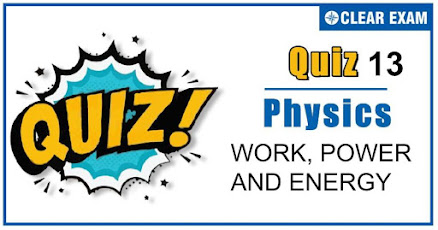## [LATEST]\$type=sticky\$show=home\$rm=0\$va=0\$count=4\$va=0

As per analysis for previous years, it has been observed that students preparing for NEET find Physics out of all the sections to be complex to handle and the majority of them are not able to comprehend the reason behind it. This problem arises especially because these aspirants appearing for the examination are more inclined to have a keen interest in Biology due to their medical background. Furthermore, sections such as Physics are dominantly based on theories, laws, numerical in comparison to a section of Biology which is more of fact-based, life sciences, and includes substantial explanations. By using the table given below, you easily and directly access to the topics and respective links of MCQs. Moreover, to make learning smooth and efficient, all the questions come with their supportive solutions to make utilization of time even more productive. Students will be covered for all their studies as the topics are available from basics to even the most advanced. .

Q1. A mass of 10 g moving with a velocity of 100 cm/s strikes a pendulum bob of mass 10 g. The two masses stick together. The maximum height reached by the system now is g=10 m/s2
•  Zero
•  5 cm
•  2.5 cm
•  1.25 cm
Solution
Initially mass 10 gm moves with velocity 100 cm/s ∴Initial momentum =10×100=1000(gm×cm)/sec After collision system moves with velocity vsys. then Final momentum =(10+10)×vsys. By applying in conservation of momentum 1000=20×vsys. ⇒vsys.)=50 cm/s If system rises upto height h then h=vsys.2/2g=(50×50)/(2×1000)=2.5/2=1.25 cm

Q2. It is easier to draw up a wooden block along an inclined plane than to haul it vertically, principally because
•  The friction is reduced
•  The mass becomes smaller
•  Only a part of the weight has to be overcome
•  ‘g’ becomes smaller
Solution
Opposing force in vertical pulling =mg But opposing force on an inclined plane is mg sin⁡θ, which is less than mg

Q3. A particle acted upon by constant forces 4i ̂+j ̂-3j ̂ and 3i ̂+j ̂-k ̂is displaced from the point i ̂+2j ̂+3k ̂ to the point 5i ̂+4j ̂+k ̂ .The total work done by the forces in SI unit is
•  20
•  40
•  50
•  30
Solution
Work done,W=F∙ds=F1+F2∙s2-s1 ={(4i ̂+ j ̂-3(k)) ̂+(3i ̂+j ̂-k ̂ ) } {(5i ̂+4j ̂+k ̂ )-(i ̂+2j ̂+3k ̂ ) } =(7i ̂+2j ̂-4k ̂ ).(4i ̂+2j ̂-2k ̂ ) =28+4+8=40 J

Q4. The power of a water pump is 200 kW. If g=10ms-2, then the amount of water it can raise in 1 min to a height of 10 m is
•  2000 L
•  1000 L
•  100 L
•  1200 L
Solution
P= mgh/t m=Pt/gh=(200×60)/(10×10)=1200 L

Q5. A sphere of mass m moving with a constant velocity u hits another stationary sphere of the same mass. If e is the coefficient of restitution, then the ratio of the velocity of two spheres after collision will be
•  (1-e)/(1+e)
•  (1+e)/(1-e)
•  (e+1)/(e-1)
•  (e-1)/(e+1) t2
Solution
(1-e)/(1+e)

Q6. A bullet of mas m moving with velocity v strikes a block of mass M at rest and gets embedded into it. The kinetic energy of the composite block will be
•  1/2 mv2×m/((m+M))
•  1/2 mv2×M/((m+M))
• 1/2 mv2×((M+m))/((M))
•  1/2 Mv2×m/((m+M))
Solution
By conservation of momentum, mv+M×0=(m+M)V Velocity of composite block V=(m/(m+M))v K.E. of composite block =1/2 (M+m) V2 =1/2 (M+m) (m/(M+m))2 v2=1/2 mv2 (m/(m+M))

Q7. An α-particle of mass msuffers one dimensional elastic collision with a nucleus of unknown mass. After the collision the α-particle is scattered directly backward losing 75% of its kinetic energy .then the mass of the nucleus is
•  m
•  2 m
•  3 m
•  3/2 m
Solution
1/2 m1 u12-1/2 m1 v12=75/100×1/2 m1 u12 Or u12-v12=3/4 u12 or v1=1/2 u1 .............(i) Now v1=m2-m1 u1/m1+m2….(ii) Thus, 1/2 u1=m2-m1 u1/m1+m2 or m2=3m1=3m

Q8. A spring gun of spring constant 90 Ncm-1is compressed 12 cm by a ball of mass 16 g. If the trigger is pulled, the velocity of the ball is
•  50 ms-1
•  T9 ms-1
•  40 ms-1
•  90ms-1
Solution
Kinetic energy of ball=potential energy of spring i.e.,B 1/2 mv2=1/2 kx2 ∴ 16×10-3×v2=90/10-2 ×12×10-22 Or v2=90×144×10-4/10-2×16×10-3 Or v=90ms-1

Q9. If momentum is increased by 20%, then kinetic energy increases by
•  48%
•  44%
•  40%
•  36%
Solution
Kinetic energy, K=P2/2m Where P is the momentum and m is the mass. When momentum is increased by 20%, then ρ'=P+20/100 P=1.2P ∴K'=1.2P2/2m=1.44P2/2m=1.44K K'=K+0.44K ⇒ K'-K)/K=0.44 Percentage increase in kinetic energy is K'-K/K×100=0.44×100=44%

Q10. A particle falls from a height h upon a fixed horizontal plane and rebounds. If e is the coefficient of restitution, the total distance travelled before rebounding has stopped is
•  h1+e2/1-e2
•  h1+e2/1-e2
•  h/2 1-e2/1+e2
• h/2 1+e2/1-e2
Solution

Particle falls from height h then formula for height covered by it in nth rebound is given by hn=he2n Where e= coefficient of restitution, n= No. of rebound Total distance travelled by particle before rebounding has stopped H=h+2h1+2h2+2h3+2h4+⋯ =h+2he2+2he4+2he6+2he8+⋯ =h+2he2+e4+e6+e8+⋯) =h+2hE2/1-e2=h1+2e2/1-e2=h1+e2/1-e2## Want to know more

Please fill in the details below:

## Latest NEET Articles\$type=three\$c=3\$author=hide\$comment=hide\$rm=hide\$date=hide\$snippet=hide

Name

ltr
item
BEST NEET COACHING CENTER | BEST IIT JEE COACHING INSTITUTE | BEST NEET, IIT JEE COACHING INSTITUTE: Work,Power And Energy Quiz 13
Work,Power And Energy Quiz 13